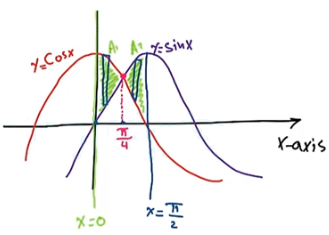Need Help?

Subscribe to Calculus B

###### \${selected_topic_name}
• Notes

Find the area of the region bounded by the curves
$y=\sin (x), y=\cos (x), x=0, x=\frac{\pi}{2}$$A \equiv A_{1}+A_{2}$

$=\int_{0}^{\frac{\pi}{4}}(\cos (x)-\sin (x)) d x+\int_{\frac{\pi}{4}}^{\frac{\pi}{2}}(\sin (x)-\cos x) d x$

$=[\sin (x)+\cos (x)]_{0}^{\frac{\pi}{4}}+[-\cos (x)-\sin (x) ]_{\frac{\pi}{4}}^{\frac{\pi}{2}}$

$=\left[\sin \frac{\pi}{4}+\cos \frac{\pi}{4}-(\sin (0)+\cos (0))\right]$

$+\left[-\cos \frac{\pi}{2}-\sin \frac{\pi}{2}-\left(-\cos \frac{\pi}{4}-\sin \frac{\pi}{4}\right)\right]$

$=\left[\frac{1}{\sqrt{2}}+\frac{1}{\sqrt{2}}-(0+1)\right]+\left[-0-1-\left(-\frac{1}{\sqrt{2}}-\frac{1}{\sqrt{2}}\right)\right]$

$=\left[\frac{2}{\sqrt{2}}-1\right]+\left[-1+\frac{2}{\sqrt{2}}\right]=\frac{2}{\sqrt{2}}-1-1+\frac{2}{\sqrt{2}}=\frac{4}{\sqrt{2}}-2=2 \sqrt{2}-2$